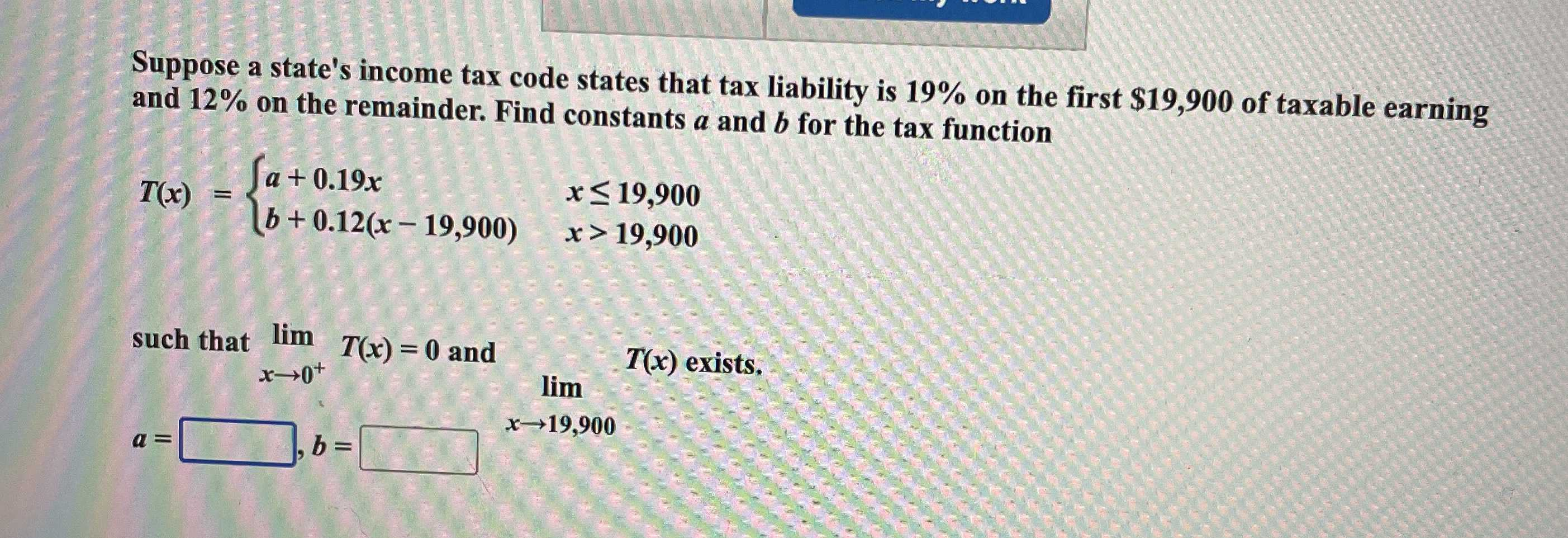### Still have math questions?

Algebra
QuestionSuppose a state's income tax code states that tax liability is $$19 \%$$ on the first $$\ 19,900$$ of taxable earning and $$12 \%$$ on the remainder. Find constants $$a$$ and $$b$$ for the tax function

$$T ( x ) = \left\{ \begin{array} { l r } { a + 0.19 x } \ \ { x \leq 19,900 }\\ { b + 0.12 ( x - 19,900 ) } \ \ { x > 19,900 } \end{array} \right.$$ such that $$\lim _ { x \rightarrow 0 ^ { + } } T ( x ) = 0$$ and $$\lim _ { x \rightarrow 19,900 } T ( x )$$ exists.$$a = \square , b = \square$$